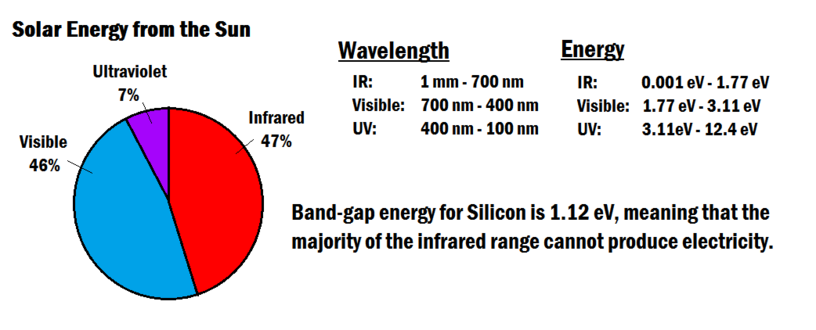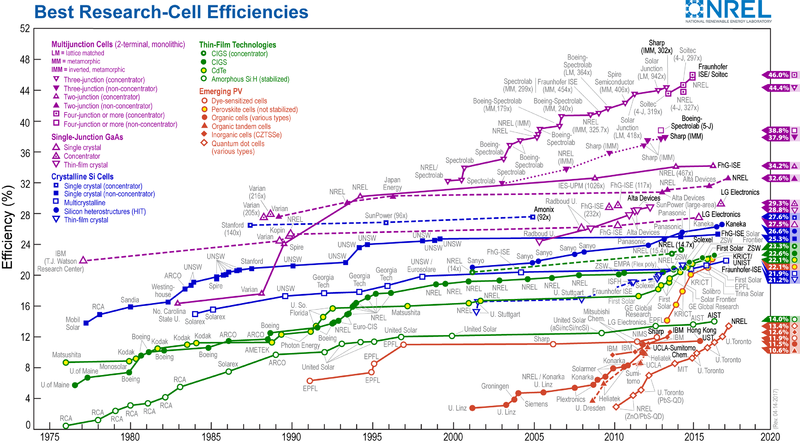# Solar cell efficiency

Efficiency is the comparison of energy output to energy input of a given system. For solar photovoltaic (PV) cells, this means the ratio of useful electrical energy they produce to the amount of solar energy incident on the cell under standardized testing conditions. Although some experimental solar cells have achieved efficiencies of close to 50%, most commercial cells are below 30%. Unlike the carnot efficiency which limits the thermal efficiency of heat engines, the efficiency of solar cells is limited by something called the "band gap energy".

## Band Gap Energy

The physics of semiconductors requires a minimum amount of energy to remove an electron from a crystal structure, known as the band gap energy. For solar cells, this energy is provided by particles of light called photons, which are tiny packets of electromagnetic radiation released from the Sun. Sunlight contains a wide spectrum of photons with different wavelengths and energy. If a photon strikes a solar cell and has less energy than the band gap, it gets absorbed as thermal energy. If it has sufficient energy it has a chance of "knocking" an electron loose and producing an electrical current. For silicon, the band gap energy is 1.12 electron volts. The longest wavelength (which corresponds to the lowest energy) that is capable of removing an electron is 1.1 µm. From Figure 2 below it can be seen that the visible light and ultraviolet light have sufficient energy, but a good portion of infrared light cannot be used. This means that ~1/4 of the light from the Sun is not sufficient enough to create electricity.Figure 2. The solar energy received by the Earth, and the corresponding energies of its photons. Efficiency of a PV cell is largely effected by the amount of incoming light that can cause current to flow.

Even from the light that can be absorbed, there is still a problem. Any energy above the band-gap energy will be transformed into heat. This also cuts the efficiency because that heat energy is not being used for any useful task. Of the electrons that are made available, not all of them will actually make it to the metal contact and generate electricity. This is because some of them will not be accelerated sufficiently by the voltage inside the semiconductor. Because of the reasons listed, the theoretical efficiency of silicon PV cells is about 33%.

## Increasing efficiency

There are ways to improve the efficiency of PV cells, all of which come with an increased cost. One way is to decrease the number of semiconductor impurities and crystal structure deformations. This can be achieved through the production of monocrystalline, or "single-crystal" cells. A more pure and uniform cell has a higher chance of interacting with incoming photons. Another method is to use a more efficient semiconducting material such as Gallium Arsenide. Although it's much more rare and expensive than silicon, gallium arsenide has an optimal band-gap of 1.4 electron volts, allowing for a higher percentage of the Sun's energy to be harnessed. Multiple layers of semiconductor material called p-n junctions can also be used to increase cell efficiency. These multi-junction cells harness energy from multiple sections of the solar spectrum as each junction has a different band gap energy. Efficiency can also be increased through concentrated photovoltaics. This method involves concentrating the Sun's energy through various methods to increase the intensity of energy hitting the solar cell. For more information about the different types of solar cells, click here. Figure 3 below shows the evolution of solar cell technologies and efficiencies from 1976 to 2017.Figure 3. Conversion efficiencies of best research solar cells worldwide for various photovoltaic technologies since 1976.